Study Guide

# Kinetics - Plotting Kinetics Experiments

## Plotting Kinetics Experiments

### Get your Graph On

y = mx + b. The formula for the boring old line. Chemists revere the line in the same way that dogs adore chew toys. Why, you ask? Because chemists like to simplify things, and let's face it, graphs of wildly shaped curves are confusing.

On top of that, lines are easy to work with because the math is straightforward. If you ever asked, "Why do I have to learn algebra anyway?" well, here is your answer.

When it comes to kinetics, the line is the end goal when we're plotting our experimental data. If our plot is linear, bingo, we win. We've confirmed the rate law for a given reactant. If not, then something went wrong.

There's a lot of math that we've omitted here, specifically regarding how the equations were derived. All the background math isn't a bad thing to know, but a full mathematical treatment isn't really necessary either.

The math for different order reactions are pretty different from each other, so we're going to look at them individually, one order at a time. If you need to do a yoga headstand to replenish the blood flow to your brain in between formulas, we won't judge. In fact, we had to just that to figure this business out.

### It Makes Zero Sense, But Not Like That

For zero-order reactions, the following are true.

1. The Rate Law is Rate = k[A]0

A plot of concentration vs. time yields a straight line. The "Kids in the Bed" example from the previous section shows just that. At a rate of 3 kids falling out of bed per minute, after 3 minutes, we have nine bruised children on the floor and one triumphant brat left in bed to gloat.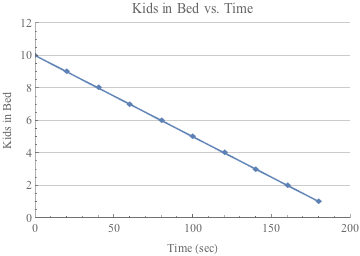2. Half-life: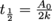For zero-order reactions, the half-life, or the time it will take for half of the reactants (in this case, kids) to reach 50%, is represented by the formula above, which also yields a straight line.

Those are our two formulas for zero-order reactions. To get us ready for first- and second-order reactions, check out the graphs below. The first is a plot of [A] vs t, the second is a plot of ln[A] vs. t, and the third is a plot of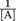. For zero-order reactions, the only plot that gives a straight line is [A] vs t. Remember that, because it'll be different for the other orders.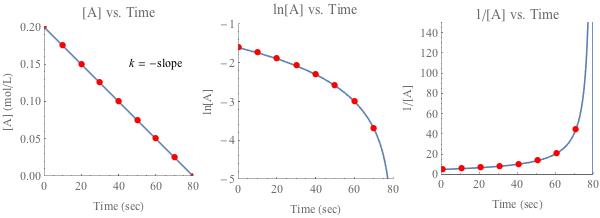### Second, Our First Order of Business

For first-order reactions, the following are true.

1. The Rate Law is Rate = k[A]1

2. Half-life: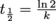3. A plot of ln[A] vs. time yields a straight line.

Once again check out our three lovely graphs below. For first-order reactions, which one of our choices gives a straight line?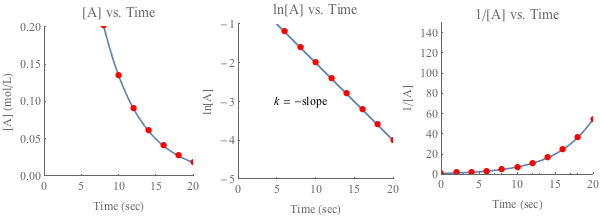It's the plot of ln[A] vs t, there in the middle.

### Wait a Second

For second-order reactions, the following are true.

1. The Rate Law is Rate = k[A]2

2. Half-life: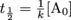3. A plot of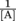vs. time yields a straight line.

It's time to find the straight line again. You'll find that only in a plot ofvs t for second-order reactions.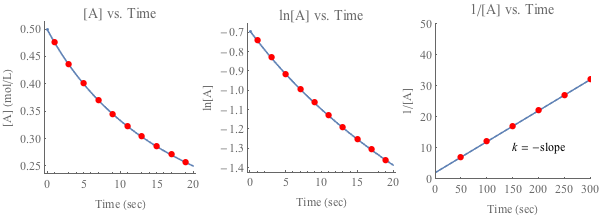### So, What's the Point?

Here's the upshot to all of this. If we know [A] for a reaction at various points in time, but we don't know the order of the reaction, we have a way of finding out the order. All we do is make these three graphs and see which one makes a straight line.

Holy smokes, math is too smart for its own good sometimes.